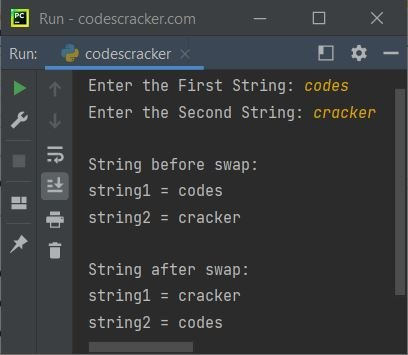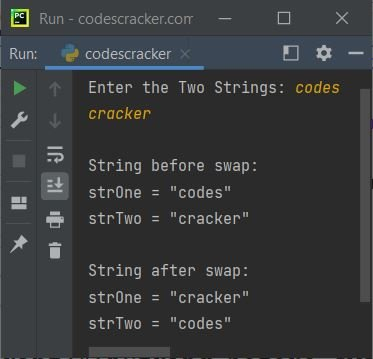# Python Program to Swap Two Strings

This article covers a program in Python, that swaps two strings, entered by user at run-time of the program.

## Swap Two Strings in Python

The question is, write a Python program to swap two given strings. The program given below is its answer:

```print("Enter the First String: ", end="")
string1 = input()
print("Enter the Second String: ", end="")
string2 = input()

print("\nString before swap:")
print("string1 =", string1)
print("string2 =", string2)

temp = string1
string1 = string2
string2 = temp

print("\nString after swap:")
print("string1 =", string1)
print("string2 =", string2)```

The snapshot given below shows the sample run of above Python program, with user input codes and cracker as two strings to swap:The above program can also be created in this way:

```print("Enter the Two Strings: ", end="")
strOne = input()
strTwo = input()

print("\nString before swap:")
print("strOne = \"", strOne, "\"", sep="")
print("strTwo = \"", strTwo, "\"", sep="")

x = strOne
strOne = strTwo
strTwo = x

print("\nString after swap:")
print("strOne = \"", strOne, "\"", sep="")
print("strTwo = \"", strTwo, "\"", sep="")```

Here is its sample run with again same user input as of previous sample run:#### Same Program in Other Languages

Python Online Test

« Previous Program Next Program »